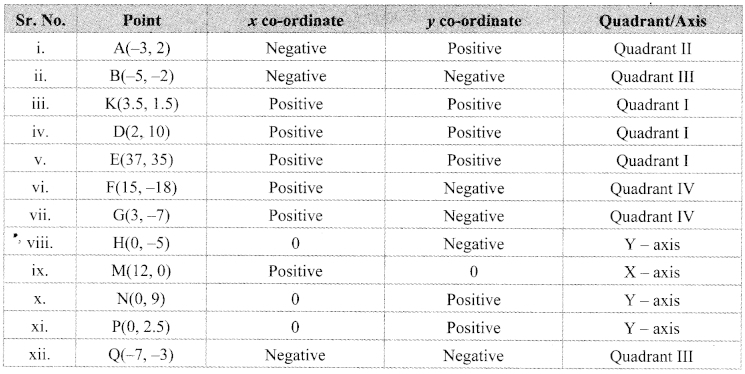# Maharashtra Board 9th Class Maths Part 2 Practice Set 7.1 Solutions Chapter 7 Co-ordinate Geometry

Balbharti Maharashtra State Board Class 9 Maths Solutions covers the Practice Set 7.1 Geometry 9th Class Maths Part 2 Answers Solutions Chapter 7 Co-ordinate Geometry.

## Practice Set 7.1 Geometry 9th Std Maths Part 2 Answers Chapter 7 Co-ordinate Geometry

Question 1.
State in which quadrant or on which axis do the following points lie.
i. A(-3, 2)
ii. B(-5, -2)
iii. K(3.5, 1.5)
iv. D(2, 10)
V. E(37, 35)
vi. F(15, -18)
vii. G(3, -7)
viii. H(0, -5)
ix. M(12, 0)
x. N(0, 9)
xi. P(0, 2.5)
xii. Q(-7, -3)
Solution:Question 2.
In which quadrant are the following points?
i. whose both co-ordinates are positive.
ii. whose both co-ordinates are negative.
iii. whose x co-ordinate is positive and the y co-ordinate is negative.
iv. whose x co-ordinate is negative and y co-ordinate is positive.
Solution:

Question 3.
Draw the co-ordinate system on a plane and plot the following points.
L(-2, 4), M(5, 6), N(-3, -4), P(2, -3), Q(6, -5), S(7, 0), T(0, -5)
Solution:Maharashtra Board Class 9 Maths Chapter 7 Co-ordinate Geometry Practice Set 7.1 Intext Questions and Activities

Question 1.
Plot the points R(-3,-4), S(3,-l) on the same co-ordinate system. (Textbook pg. no. 93)
Steps for plotting the points:
i. Draw X-axis and Y-axis on the plane. Show the origin.
ii. Draw a line parallel to Y-axis at a distance of 3 units in the -ve direction of X-axis.
iii. Draw another line parallel to X-axis at a distance of 4 units in the -ve direction of Y-axis.
iv. Intersection of these lines is the point R (-3, -4).
v. The point S can be plotted in the same manner.Scroll to Top